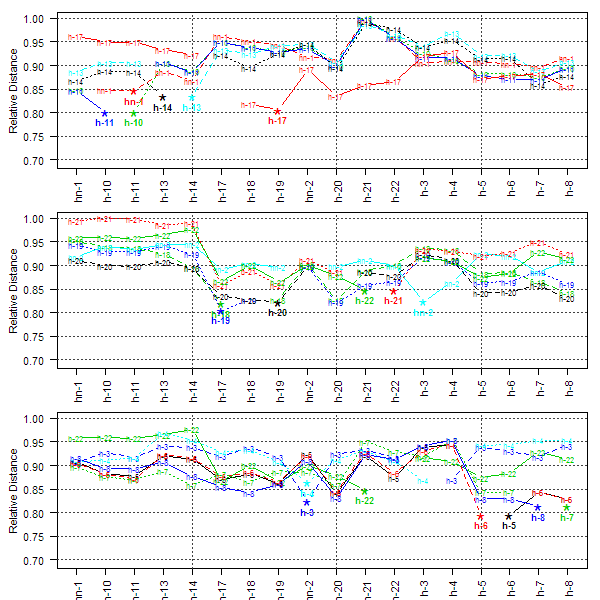plot_distance_graph {aqp} R Documentation

## Between Individual Distance Plot

### Description

Plot pair-wisee distances between individuals as line segments.

### Usage

```plot_distance_graph(D, idx = 1:dim(as.matrix((D))))
```

### Arguments

 `D` distance matrix, should be of class 'dist' or compatible class `idx` an integer sequence defining which individuals should be compared

### Details

By default all individuals are plotting on the same axis. When there are more than about 10 individuals, the plot can become quite messy. See examples below for ideas.

### Value

No value is returned.

### Author(s)

Dylan E Beaudette

### References

http://casoilresource.lawr.ucdavis.edu/

`sp2`, `profile_compare`

### Examples

``````data(sp2)

d <- profile_compare(sp2, vars=c('prop','field_ph','hue','value'),
max_d=100, k=0.01, sample_interval=5)
``````
``````##        id prop field_ph hue value
## 1   hon-1    0       22  22    22
## 4  hon-13    0        8   8     8
## 8  hon-19    0       17  17    17
## 9   hon-2    0       17  17    17
## 10 hon-20    0       12   0     0
## 17  hon-7    0       30  30    30
## 18  hon-8    0       12  12    12
``````
``````par(mfcol=c(3,1), mar=c(2.5,4.5,1,1))
plot_distance_graph(d, idx=1:6)
plot_distance_graph(d, idx=7:12)
plot_distance_graph(d, idx=12:18)
``````[Package aqp version 1.9.1 Index]